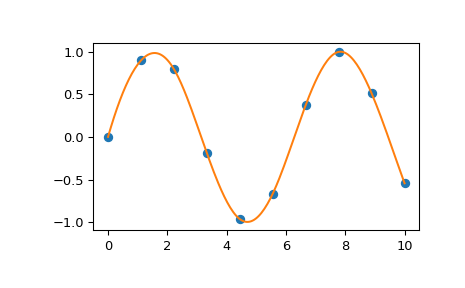# scipy.interpolate.splrep¶

scipy.interpolate.splrep(x, y, w=None, xb=None, xe=None, k=3, task=0, s=None, t=None, full_output=0, per=0, quiet=1)[source]

Find the B-spline representation of 1-D curve.

Given the set of data points (x[i], y[i]) determine a smooth spline approximation of degree k on the interval xb <= x <= xe.

Notes

See splev for evaluation of the spline and its derivatives. Uses the FORTRAN routine curfit from FITPACK.

The user is responsible for assuring that the values of x are unique. Otherwise, splrep will not return sensible results.

If provided, knots t must satisfy the Schoenberg-Whitney conditions, i.e., there must be a subset of data points x[j] such that t[j] < x[j] < t[j+k+1], for j=0, 1,...,n-k-2.

This routine zero-pads the coefficients array c to have the same length as the array of knots t (the trailing k + 1 coefficients are ignored by the evaluation routines, splev and BSpline.) This is in contrast with splprep, which does not zero-pad the coefficients.

References

Based on algorithms described in [R113], [R114], [R115], and [R116]:

 [R113] (1, 2) P. Dierckx, “An algorithm for smoothing, differentiation and integration of experimental data using spline functions”, J.Comp.Appl.Maths 1 (1975) 165-184.
 [R114] (1, 2) P. Dierckx, “A fast algorithm for smoothing data on a rectangular grid while using spline functions”, SIAM J.Numer.Anal. 19 (1982) 1286-1304.
 [R115] (1, 2) P. Dierckx, “An improved algorithm for curve fitting with spline functions”, report tw54, Dept. Computer Science,K.U. Leuven, 1981.
 [R116] (1, 2) P. Dierckx, “Curve and surface fitting with splines”, Monographs on Numerical Analysis, Oxford University Press, 1993.

Examples

>>> import matplotlib.pyplot as plt
>>> from scipy.interpolate import splev, splrep
>>> x = np.linspace(0, 10, 10)
>>> y = np.sin(x)
>>> spl = splrep(x, y)
>>> x2 = np.linspace(0, 10, 200)
>>> y2 = splev(x2, spl)
>>> plt.plot(x, y, 'o', x2, y2)
>>> plt.show()#### Previous topic

scipy.interpolate.make_lsq_spline

#### Next topic

scipy.interpolate.splprep